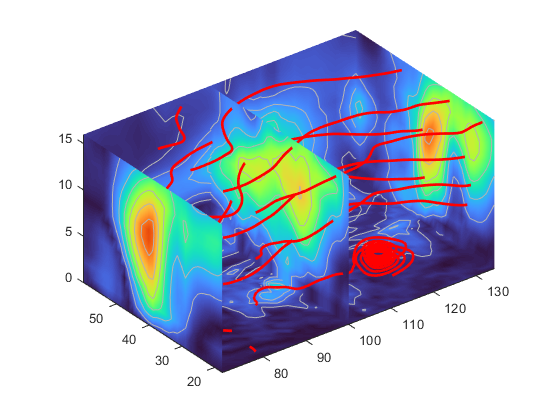Main Content

## Display Streamlines Using Vector Data

### Wind Mapping Data

The MATLAB® vector data set called `wind` represents air currents over North America. This example uses a combination of techniques:

• Stream lines to trace the wind velocity

• Slice planes to show cross-sectional views of the data

• Contours on the slice planes to improve the visibility of slice-plane coloring

### 1. Determine the Range of the Coordinates

Load the data and determine minimum and maximum values to locate the slice planes and contour plots (`load`, `min`, `max`).

```load wind xmin = min(x(:)); xmax = max(x(:)); ymax = max(y(:)); zmin = min(z(:));```

### 2. Add Slice Planes for Visual Context

Calculate the magnitude of the vector field (which represents wind speed) to generate scalar data for the `slice` command. Create slice planes along the x-axis at `xmin`, `100`, and `xmax`, along the y-axis at `ymax`, and along the z-axis at `zmin`. Specify interpolated face coloring so the slice coloring indicates wind speed, and do not draw edges (`sqrt`, `slice`, `FaceColor`, `EdgeColor`).

```wind_speed = sqrt(u.^2 + v.^2 + w.^2); hsurfaces = slice(x,y,z,wind_speed,[xmin,100,xmax],ymax,zmin); set(hsurfaces,'FaceColor','interp','EdgeColor','none') colormap turbo```

### 3. Add Contour Lines to the Slice Planes

Draw light gray contour lines on the slice planes to help quantify the color mapping (`contourslice`, `EdgeColor`, `LineWidth`).

```hcont = ... contourslice(x,y,z,wind_speed,[xmin,100,xmax],ymax,zmin); set(hcont,'EdgeColor',[0.7 0.7 0.7],'LineWidth',0.5)```

### 4. Define the Starting Points for Stream Lines

In this example, all stream lines start at an x-axis value of 80 and span the range 20 to 50 in the y-direction and 0 to 15 in the z-direction. Save the handles of the stream lines and set the line width and color (`meshgrid`, `streamline`, `LineWidth`, `Color`).

```[sx,sy,sz] = meshgrid(80,20:10:50,0:5:15); hlines = streamline(x,y,z,u,v,w,sx,sy,sz); set(hlines,'LineWidth',2,'Color','r') ```

### 5. Define the View

Set up the view, expanding the z-axis to make it easier to read the graph (`view`, `daspect`, `axis`).

```view(3) daspect([2,2,1]) axis tight```See `coneplot` for an example of the same data plotted with cones.

Download ebook